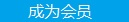您可以捐助，支持我们的公益事业。 1元 10元 50元 认证码：必填求知 文章 文库 Lib 视频 Code iProcess 课程 角色 咨询 工具 火云堂 讲座吧 建模者要资料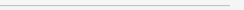订阅捐助
Python大数据处理案例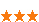1096 次浏览     评价： 好 中 差
2018-8-7

 编辑推荐: 来源于cnblogs，介绍了利用决策树分类，利用随机森林预测， 利用对数进行fit，和exp函数还原等。lubridate包拆解时间 | POSIXlt

https://www.kaggle.com/c/bike-sharing-demand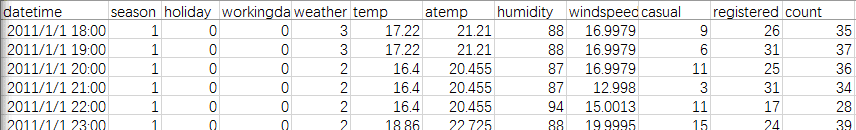1.png

 library (lubridate) library (randomForest) library (readr) setwd ("E:") data <-read_csv ("train.csv") head (data)

 test\$registered=0 test\$casual=0 test\$count=0 data<-rbind(train,test)

 data\$hour1<-substr (data\$datetime,12,13) table(data\$hour1)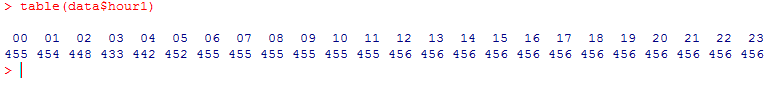6-hour1.png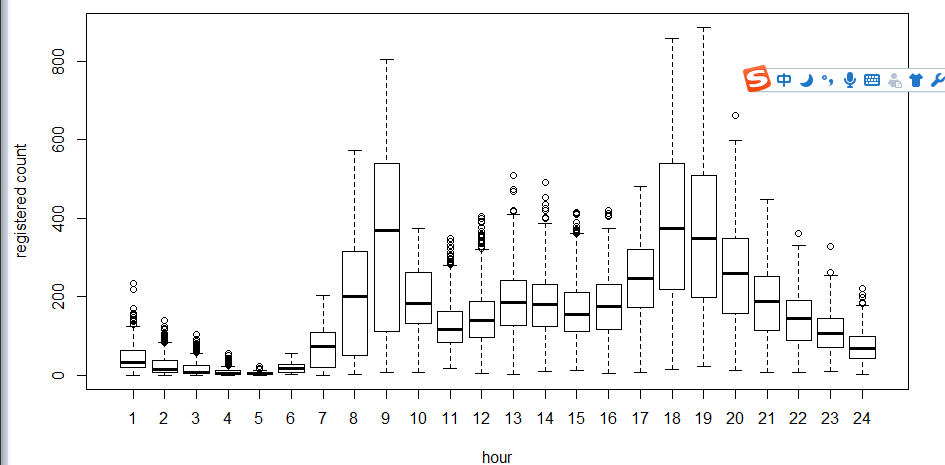5-hour-regestered.png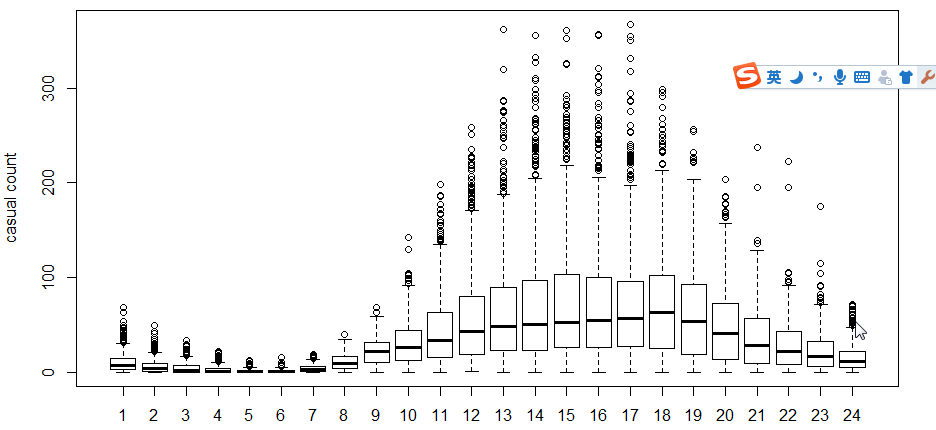5-hour-casual.png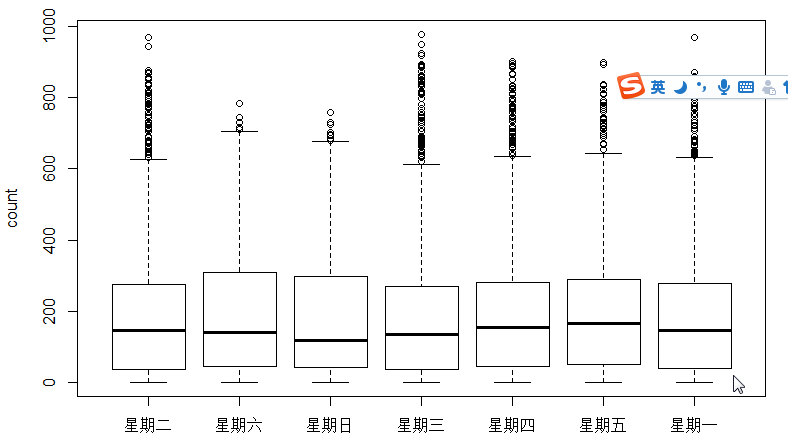4-boxplot-day.png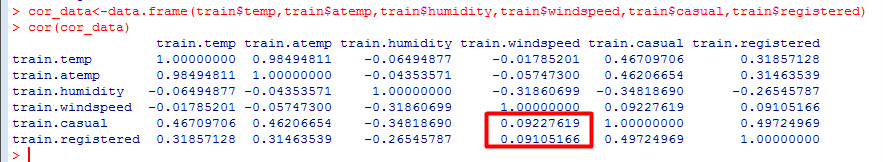cor.png

 train\$hour1<-as.integer(train\$hour1) d<-rpart (registered~hour1,data=train) rpart .plot(d) >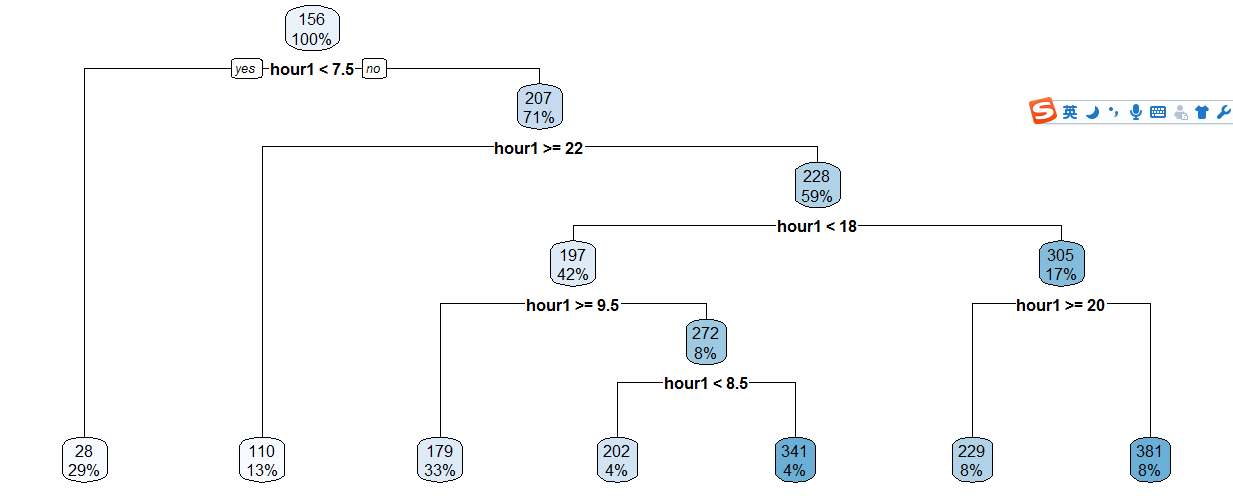3-raprt-hour1.png

 train\$hour1 <-as.integer(train\$hour1) data\$dp_reg=0 data\$dp_reg[data\$hour1 <7.5]=1 data\$dp_reg[data\$hour1> =22]=2 data\$dp_reg[data\$hour1 >=9.5 & data\$hour1<18]=3 data\$dp_reg[data\$hour1> =7.5 & data\$hour1<18]=4 data\$dp_reg[data\$hour1> =8.5 & data\$hour1<18]=5 data\$dp_reg[data\$hour1> =20 & data\$hour1<20]=6 data\$dp_reg[data\$hour1> =18 & data\$hour1<20]=7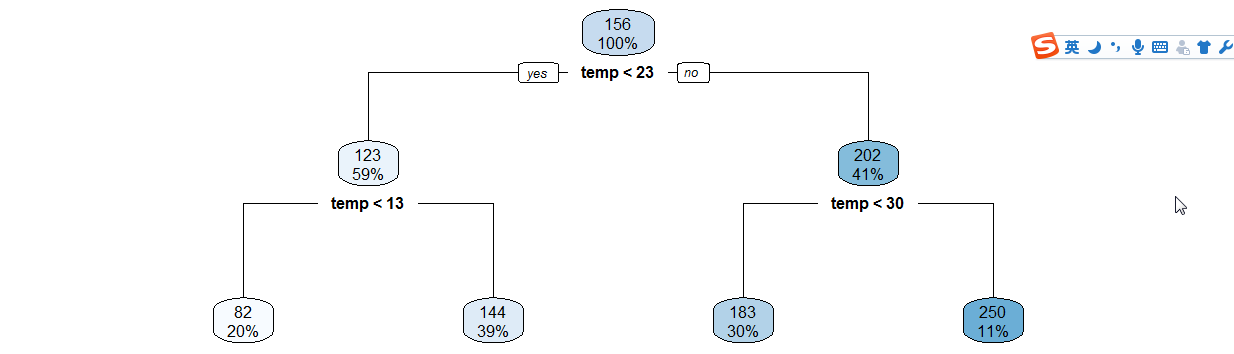3-raprt-temp.png

 data\$year_part=0 data\$month <-month(data\$datatime) data\$year_part [data\$year=='2011']=1 data\$year_part [data\$year=='2011' & data \$month>3] = 2 data\$year_part[data\$year=='2011' & data \$month>6] = 3 data\$year_part[data\$year=='2011' & data \$month>9] = 4 data\$day_type="" data\$day _type [data\$holiday ==0 & data\$workingday==0] ="weekend" data\$day_type[data\$holiday==1] ="holiday" data\$day_type[data\$holiday ==0 & data\$workingday==1] ="working day" data\$weekend=0 data\$weekend [data\$day= ="Sunday"|data\$day= =" Saturday "] =1

ntree指定随机森林所包含的决策树数目，默认为500，通常在性能允许的情况下越大越好；

mtry指定节点中用于二叉树的变量个数，默认情况下数据集变量个数的二次方根（分类模型）或三分之一（预测模型）。一般是需要进行人为的逐次挑选，确定最佳的m值―摘自datacruiser笔记。这里我主要学习，所以虽然有10000多数据集，但也只定了500。就这500我的小电脑也跑了半天。

 train<-data set.seed (1234) train\$logreg <-log(train\$registered+1) test\$logcas <-log(train\$casual+1) fit1 <-randomForest (logreg~hour1+ workingday + day + holiday + day_ type+ temp_reg+ humidity + atemp + windspeed + season+ weather+ dp_ reg+ weekend + year+year _part ,train ,importance = TRUE , ntree = 250) pred1 <-predict (fit1,train) train \$logreg <-pred1

 train\$registered<-exp(train\$logreg)-1 train\$casual<-exp(train\$logcas)-1 train\$count<-test\$casual+train\$registered

 train2<-train[as.integer (day(data\$datetime))> = 20 ,] submit_final <-data.frame (datetime= test\$ datetime ,count= test\$count) write.csv(submit _final,"submit_ final.csv ", row .names = F)1096 次浏览  评价: 好 中 差订阅捐助
 相关文章 手机软件测试用例设计实践 手机客户端UI测试分析 iPhone消息推送机制实现与探讨 Android手机开发（一）
 相关文档 Android_UI官方设计教程 手机开发平台介绍 android拍照及上传功能 Android讲义智能手机开发
 相关课程 Android高级移动应用程序 Android系统开发 Android应用开发 手机软件测试每天2个文档/视频 扫描微信二维码订阅
 订阅技术月刊 获得每月300个技术资源